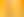It's All in the Numbers

# Stages1. Visualising Multiplication

EXPLORE

Suggested Learning Intentions

• To multiply two-digit numbers by partitioning them into smaller numbers, using a visual model as a support

Sample Success Criteria

• I can partition two-digit numbers
• I can use grids or arrays to help solve a multiplication problem
• I can model and explain my thinking using manipulatives
EXPLORE

2. Liquorice Factory

EXPLORE

Suggested Learning Intentions

• To develop an understanding and definition of prime numbers

Sample Success Criteria

• I can identify prime numbers and explain why these are prime
• I can use manipulatives to model and explain the properties of prime and composite numbers
EXPLORE

3. Daniel's Great Flight

EXPLORE

Suggested Learning Intentions

• To position and compare positive and negative numbers on a number line
• To develop strategies for adding and subtracting positive and negative numbers

Sample Success Criteria

• I can locate positive and negative numbers on a number line
• I can add and subtract positive and negative numbers
• I can use manipulatives to explain positive and negative numbers
EXPLORE

EXPLORE

Suggested Learning Intentions

• To improve fluency when working with a range of mathematical operations
• To learn how to correctly use different operations to evaluate mathematical statements

Sample Success Criteria

• I can use combinations of operations to create target numbers
• I can use manipulatives to explain and justify my thinking
EXPLORE

5. Number Puzzles

EXPLORE

Suggested Learning Intentions

• To use recognised problem-solving strategies to solve a number puzzle

Sample Success Criteria

• I can select appropriate problem-solving strategies to help me solve a number puzzle
• I can use manipulatives to explain and justify the solutions I have found
EXPLORE

6. Number Properties

EXPLORE

Suggested Learning Intentions

• To explore a range of number properties and use these properties to arrange numbers into sets
• To recognise that some pairs of sets can have common numbers, and some pairs of sets do not have common numbers

Sample Success Criteria

• I can identify properties of numbers
• I can organise numbers into sets with common properties
• I can explain my thinking using manipulatives
EXPLORE